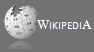Completely Solved C, C++ Programs Assignment.Quick Search Database Videos Tutorials Ebooks Forums FAQ Aboutus Household Industrial Manufacturing Service Shopping Transportation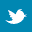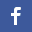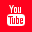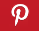### Data structure(Infix,Prefix,Postfix)-PART 2

 Filed Under: Moved to New Place

 II. INFIX, POSTFIX AND PREFIX
Consider a simple expression : A + B. This notation is called Infix Notation.
A + B in Postfix notation is A B +
As you might have noticed, in this form the operator is placed after the operands (Hence the name 'post'). Postfix is also known as Reverse Polish Notation (RPN).
Similarly for Infix, the operator is placed Inside the operands.
Likewise the equivalent expression in prefix would be + A B, where the operator precedes the operands.
So if X1 and X2 are two operands and OP is a given operator then
Infix Postfix Prefix
X1 OP X2 = X1 X2 OP = OP X1 X2
We use infix notation for representing mathematical operations or for manually performing calculations, since it is easier to read and comprehend. But for computers to perform quick calculations, they must work on prefix or postfix expressions.
Now let's take a slightly complicated expression : A + B / C
How do we convert this infix expression into postfix?
For this we need to use the BODMAS rule. (Remember?)
This rule states that each operator has its own priority and the operators with the highest priority are evaluated first. The operator priority in Descending order is BODMAS which is:
Hence, in the preceding example, B / C is evaluated first and the result is added to A.
To convert A + B / C into postfix, we convert one operation at a time. The operators with maximum priority are converted first followed by those which are placed lower in the order. Hence, A + B / C can be converted into postfix in just X steps.
0: A + B / C (First Convert B / C -> B C /)
1: A + B C / (Next Operation is A + (BC/) -> A BC/ +)
2: A B C / + (Resultant Postfix Form)
The same procedure is to be applied to convert infix into prefix except that during conversion of a single operation, the operator is placed before the two operands. Let's take the same example and convert it to Prefix Notation.
0: A + B / C (First Convert B / C -> / B C)
1: A + / B C (Next Operation is A + (/BC) -> + A /BC
2: + A / B C
Sometimes, an expression contains parenthesis like this: A + B * ( C + D )
Parenthesis are used to force a given priority to the expression that it encloses. In this case, C+D is calculated first, then multiplied to B and then added to A. Without the parenthesis, B * C would have been evaluated first.
To convert an expression with paranthesis, we first convert all expressions that are enclosed within the simple brackets like this:
[INFIX TO POSTFIX]
0: A + B * ( C + D )
1: A + B * C D +
2: A + B C D + *
3: A B C D + * +
Once an expression has been converted into postfix or prefix, there is no need to include the parenthesis since the priority of operations is already taken care of in the final expression.
IMPORTANT:
Keep in mind that converting this expression back to infix would result in the same original infix expression without the paranthesis, which would ruin the result of the expression.
Only the Prefix and Postfix forms are capable of preserving the priority of operations and are hence used for evaluating expressions in stead of infix.